# 牛顿法 防混淆 总结

wuchangjian2021-11-03 19:23:45编程学习

1） 牛顿法（最初，求的是根）

``````f(x) = f(x0)+f'(x0)(x-x0)+o(x) ----(忽略o(x) 高次项 ）
``````

`````` f(x0)+f'(x0)(x-x0) = 0    => x = x0 - f(x0) / f'(x0)
令x1=x.
``````

x1,比x0 更加接近x*（最佳值）。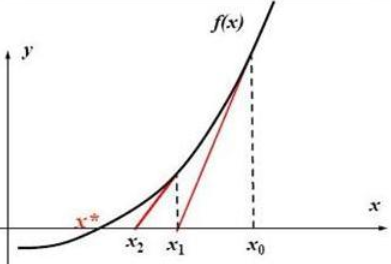x(k+1) = x(k) - f(x(k)) / f’(x(k))
（代码实现，网上太多，。。。）

2） 牛顿法（一般，求的是最小值）

``````f(x) = f(x0)+f'(x0)(x-x0)+1/2*f''(x0)(x-x0)^2+o(x) ----(忽略o(x) 高次项 ）
``````

``````f'(x)=0+f'(x0)+f"(x0)(x-x0)

``````

`````` x(k+1) = x(k) - ∇f / ∇² f
``````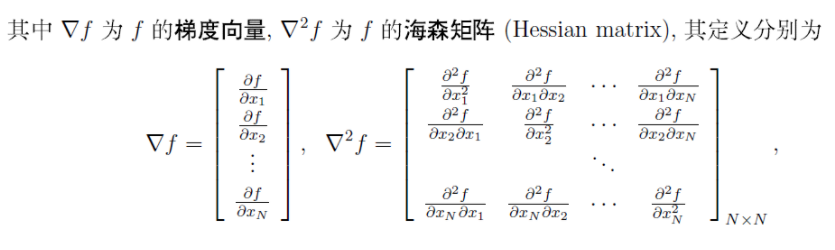3） 高斯牛顿法（一般，∇² f 不好求，所以用高斯牛顿法）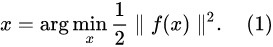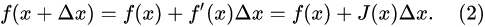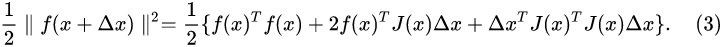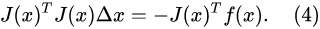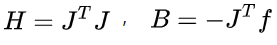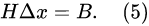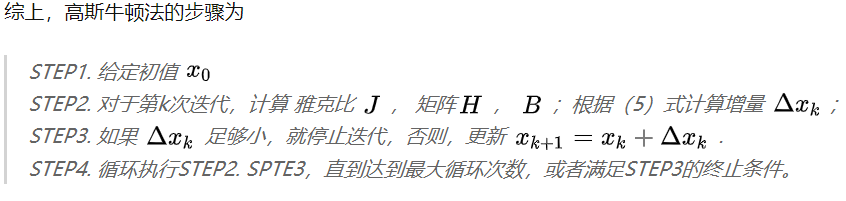...

### seoer软文推广从点到面

很多的站长都在发布外链接，但是很多的发布的外链都是不被收录的ÿ...

...

...

### [Django框架课学习笔记3]游戏创建，项目架构搭建，全局设置完毕

...

#### 发表评论◎欢迎参与讨论，请在这里发表您的看法、交流您的观点。